# PBG Microcavities in Optical Waveguides

## Introduction

In Photonic Bandgap (PBG) materials, light can be confined within a volume of the order of (λ/2n)3, where λ is the emission wavelength and n the refractive index of the material. The ability to confine light to such small volumes promises important potential applications, including resonant-cavity LED’s, zero-threshold semiconductor laser devices, and efficient erbium-doped Si light emitters.

In this application note, we simulate a PBG microcavity structure that is integrated directly into a submicron-scale silicon waveguide. An SEM image and a schematic of the PBG waveguide microcavity are shown in Fig 1. This microcavity has a resonance at λ=1.547μm. This PBG microcavity structure can easily be drawn in the RSoft CAD, as shown in Fig 2.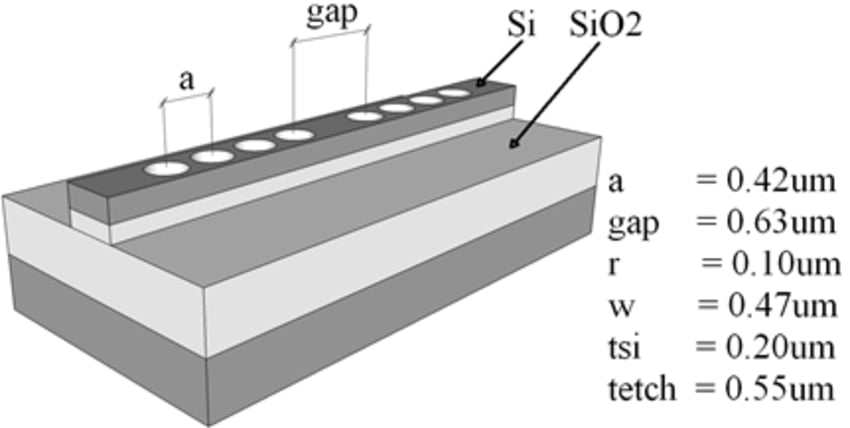Figure 1: The PBG waveguide microcavity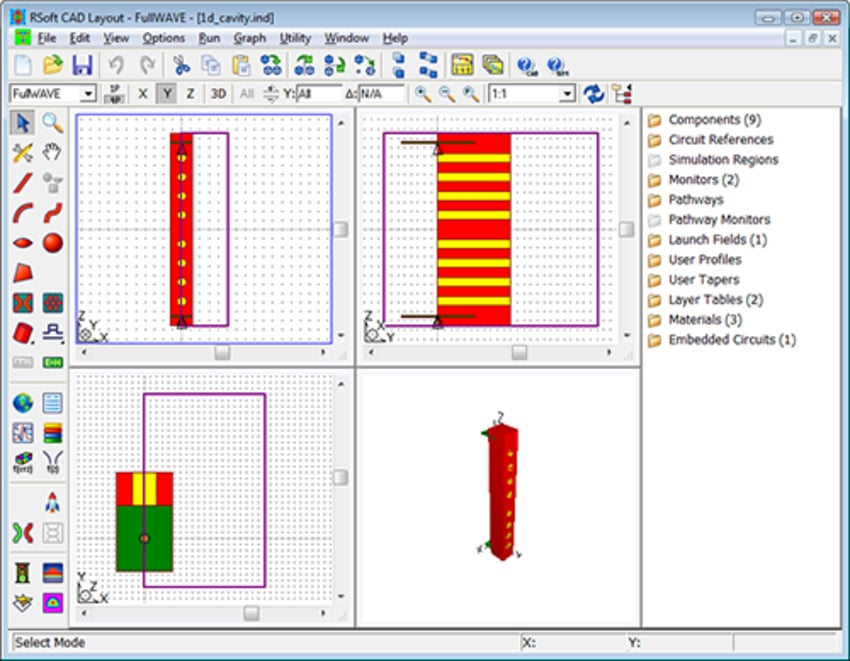Figure 2: RSoft CAD Environment™ layout of the PBG waveguide microcavity

## Simulation algorithms

RSoft's Q-Finder utility offers a robust, fast, and convenient tool for the analysis of cavity modes and Q-factors. The Q-finder utility can be used to automatically measure the Q value, mode volume, and mode profiles of a cavity structure; this automatic calculation is greatly preferred to a manual calculation of these quantities as the automatic calculation saves the user time and effort, and provides more accurate results as the automatic calculation is less subject to user error. The analysis of cavity modes and Q-factors by FullWAVE's Q-Finder tool is based on the Fast Harmonic Analysis (FHA) technique, which is discussed in detail in the RSoft FullWAVE manual.

The waveguide modes can be computed by using either RSoft's full-vector Beam Propagation Method based mode solver, or the even more rigorous FemSIM finite element mesh based mode solver. In this application note, we will use FemSIM for mode computation.

## Q-value and mode profile calculation:

First, we will use the Q-finder utility to automatically calculate the microcavity Q value and mode volume: these are important values for cavity qualifications. From the Q-Finder utility, we observe that Q = 355 and the mode volume is only 0.022; this mode is very well confined.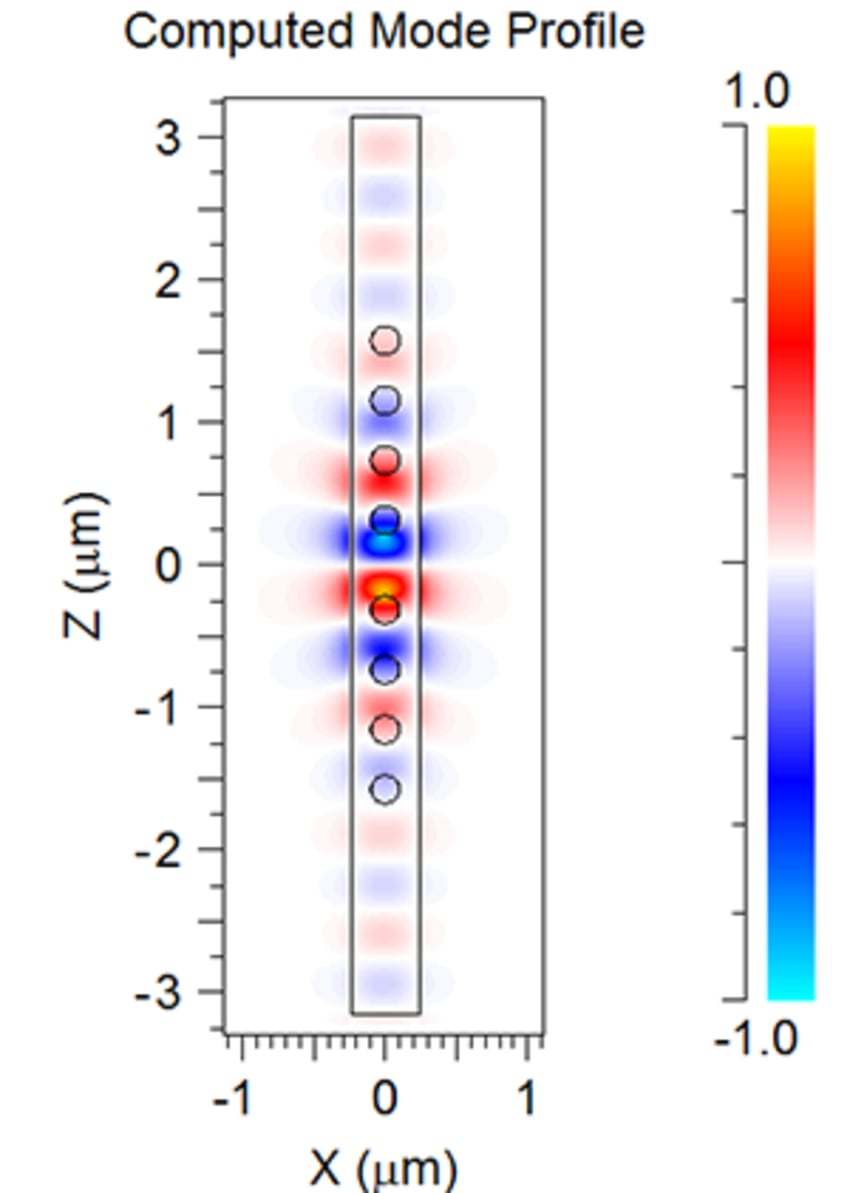Fig. 3 Cavity mode profile (right)

## Waveguide mode calculation:

The open cavity mode, as automatically calculated by the FemSIM™ mode solver, is shown in Fig. 4.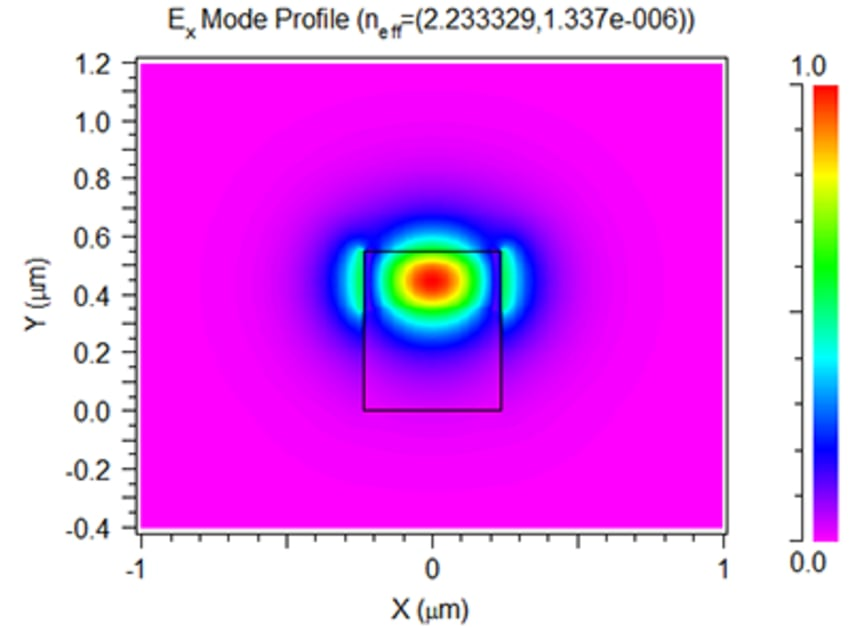Figure 4: Ex component profile of waveguide mode

## Transmission Spectrum calculation:

FullWAVE can be used to compute the transmission spectrum of this structure since the underlying algorithm behind FullWAVE, FDTD, solves Maxwell's equations directly without any physical approximations. To calculate the transmission spectrum using FullWAVE, we will use the waveguide mode that we calculated earlier as a launch excitation.

FDTD is a very rigorous algorithm, and due to this rigorousness can be computationally expensive. For an efficient simulation is therefore very important that the FDTD settings be set correctly: to streamline the design process, FullWAVE built-in settings optimizer can be used to have FullWAVE automatically select optimal FDTD time grid settings.

The computed transmission spectrum from FullWAVE is shown in Fig 5. The peak position in the bandgap is located at 1.5531μm, and matches well with the experimental results in Ref .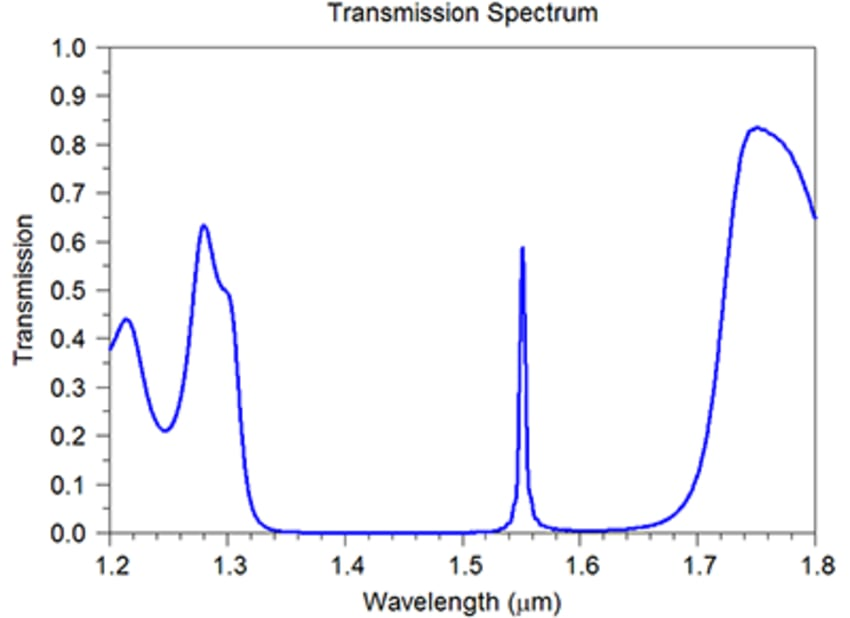Fig.5 Computed Transmission spectrum of the PBG cavity structure

## References:

1 J. S. Foresi et al, "Photonic-bandgap microcavities in optical waveguides", Nature390 1997.